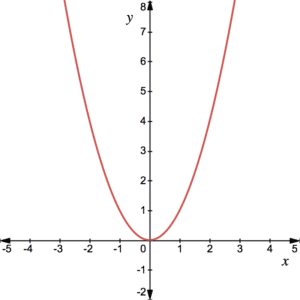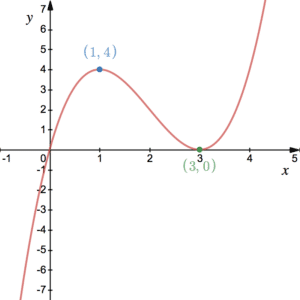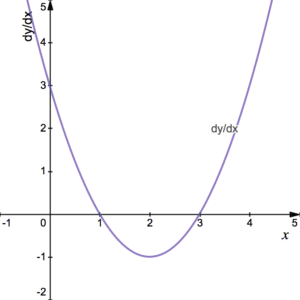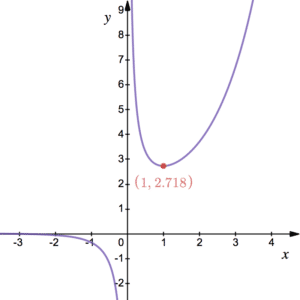# Increasing and Decreasing Functions – pos/neg gradientDifferentiation can be used to identify increasing and decreasing functions. The intervals where a function is either increasing or decreasing can then be used to sketch the curve of a derivative. Recall that upward sloping straight lines have a positive gradient whereas downward sloping straight lines have a negative gradient. The same applies to curves. Gradients on a curve are always changing but an upward sloping curve has a positive gradient and a downward sloping curve has a negative gradient.

Recall the graph of $y=x^2$. You will notice that for positive $x$, the graph has a positive gradient; for negative $x$ the graph has a negative gradient; and for $x=0$ the gradient is  0.This can be seen from the gradient function $\frac{dy}{dx}=2x$. Find out more about differentiating. $2x$ is positive when $x$ is positive, negative when $x$ is negative and 0 when $x$ is 0.

Now consider a slightly more complicated example of the cubic $y=x(x-3)^2$. Click here to see how to sketch cubics. Firstly, it can be seen from the graph that for $x<1$ the graph is increasing. At $x=1$, there is a stationary point where the gradient is 0. Next, for $x$ between 1 and 3, the graph is decreasing. There is another stationary point at $x=3$. Then the curve increases again. We can say that the cubic is a strictly increasing function on $x<1$ or $x>3$ and a strictly decreasing function on $1<x<3$. Note that the inequalities are strict.

• The function $f(x)$ is increasing on the interval $[a,b]$ if $f'(x)\geq 0$ for all x on $[a,b]$. Additionally, $f(x)$ is strictly increasing if the inequality is strict.
• The function $f(x)$ is decreasing on the interval $[a,b]$ if $f'(x)\leq 0$ for all x on $[a,b]$. Additionally, $f(x)$ is strictly decreasing if the inequality is strict.

See Examples 1 and 2. Page 21 onwards of the StudyWell Differentiation eGuide has more on increasing and decreasing functions including some exam-style questions.

## Sketching Derivatives

Consider again the graph of $y=x^2$ and its derivative $\frac{dy}{dx}=2x$. The gradient is twice the $x$-coordinate and, as we saw before, this is positive/negative/zero when $x$ is positive/negative/zero.Now consider again the graph of the cubic $y=x(x-3)^2$ above. We can attempt to sketch the graph of the derivative by first recognising that it should be quadratic. Then, recall that the stationary points are at $x=1$ and $x=3$ and so the derivative must cross the $x$-axis at these points. Finally, the derivative is negative for $1<x<3$, i.e. below the $x$-axis, and positive otherwise. It follows that the curve of the derivative is as seen here. This has been achieved without finding an expression for the derivative.

See Example 3. Note that if the original curve is speed against time, for example, the derivative will show acceleration against time. See more on this or click here for a velocity curve.

Page 27 onwards of the StudyWell Differentiation eGuide has more on sketching derivatives including some exam-style questions.

## Examples of Increasing & Decreasing Functions

Find the range of values of x for which the graph of $y=x+\frac{7}{x}$ is a decreasing function.

Explain why  $f(x)=x^3-4x^2+16x-7$ is a strictly increasing function for all $x$.The diagram shows the graph of $y=\frac{e^x}{x}$. The curve is increasing for $x>1$ and decreasing for $x<1$ except at $x=0$ where there is an asymptote. Additionally, the curve has a stationary point at $(1,e)$. Using this information, sketch the graph of  $\frac{dy}{dx}$.